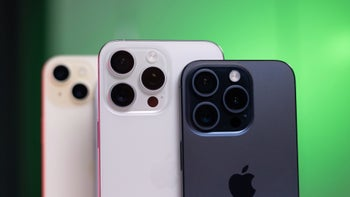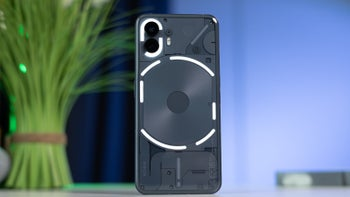# Apple iPhone X's 5.8-inch screen is actually smaller than the 5.5-inch iPhone 8 Plus display

31

The Apple iPhone X has a 5.85-inch display, the iPhone 8 Plus has a 5.5-inch screen.

So... the iPhone X has a bigger screen, right?

Wrong!

What these display measurements do not take into account is the aspect ratio of the screen, and the iPhone X has a taller and narrower display with a 2.17:1 aspect ratio and a big cutout at the top, while the iPhone 8 Plus has a 16:9 screen. This is a crucial distinction because when you actually get to calculate the screen area, you will see some surprising numbers.

Let's go ahead and do just that. Here is a look at the two displays in numbers:

• 1125 x 2436 pixels = 375 x 812 points (3x)
• 1242 x 2208 pixels = 414 x 736 points (3x)

Apple uses points as the absolute reference number, so we will also use the points number as a reference, since the difference in resolution is a topic of another discussion. Today, we only look at the sheer size of the screens.

First, we simplify things and take the point numbers to calculate the screen area without taking into account the big cutout and the curved edges of the iPhone X (we also add the iPhone 8 for more context)...

• iPhone X approximated screen area: 375 * 812 = 304,500 points
• iPhone 8 Plus real screen area: 414 * 736 = 304,704 points
• iPhone 8 real screen area: 375 * 667 = 250,125 points

So... even without taking into account those numbers, it's clear that the iPhone 8 Plus has a bigger area than the iPhone X. But how much bigger exactly?

To understand that, we need to do some further calculations. We now know the size of the iPhone X cutout in exact point dimensions as well as the radii of the curves. Let's roll up our sleeves and do some simple maths.

## Maths time!

1. Calculating the cutout size

First, the cutout area. If it was a rectangle with no rounded corners, we would have had an area of 209 points by 30 points, or 6,270 points. But we have a rounded rectangle at the two bottom edges. Let's account for that:

We first calculate the area of a 40 by 40 square, and then take out the area of the 40-pt radius circle inside. We get the area of the four leftovers in between the square and the circle, and we have two of them. Here are the calculations:

Square area: 40 * 40 = 1,600 points
Circle area: π * 202 = 1256.6, ~1257 points
Leftovers = Square area minus Circle area, or 1600 - 1257 = 343 points. A quarter of the leftovers is 85.75, ~86 points, and we have two of them for a total of 172 points.

We now have to add the tiny edges at the top.

Again, we add two squares, this time with 12-point sides, so we have 2 * (12 * 12) = 2 * 144 points. Then, the area of each 6pt circle: π × 62 = 113 points. We only need to add that quarter of the rectangle shaped by the circle, so we take out the circle area from the rectangle area and divide by four: (144 - 113) / 4 = 31 / 4 = 7.75 points, which we round up to 8 points. We have two such quarters, so it's 2 * 8 points, or 16 points.

So! The total area of the cutout is thus: the area of the rectangle minus the edge at the bottom plus the tiny edge at the top, or 6270 points - 172 points + 16 points = 6,114 points.

2. Calculating the rounded edges

We use the same trick to calculate the rounded edges: a square with an 88-point side, with a 44pt radius circle inside it. The leftovers are the four edges. Let's calculate:

Square area: 88 * 88 = 7,744 points
Circle area: π * 442 = 6,082 points
Leftovers = Square area minus Circle area, or 7744 - 6082 = 1,662 points, we have four quarters at each side, so the we take the full 1,662 point number.

3. Calculating the total iPhone X screen area in points

First, we deduct rounded edges from screen rectangle size:
304500 points - 1662 points = 302,838 points

Secondly, we deduct the cutout at the top from the number we have just gotten:
302838 points - 6114 points = 296,724 points

And voila! We finally have the one number we need: the total screen area of the iPhone X is exactly 296,724 points. High five!

## The grand total

Let's look at the grand total of all those calculations: the screen area of the iPhone X is exactly 296,724 points. The screen area of the iPhone 8 Plus is 304,704 points.

• iPhone X real screen area: 296,724 points
• iPhone 8 Plus real screen area: 304,704 points
• iPhone 8 real screen area: 250,125 points

This means that the iPhone X has 97.38% the area of the iPhone 8 Plus, or simply put, its screen is 2.6% smaller.

And when compared to the iPhone 8 and its 4.7-inch display, the iPhone X actually has an 18.6% bigger display.

And that's how the 5.8-inch iPhone X display is smaller than the 5.5-inch iPhone 8 Plus screen.

 Display Info iPhoneX iPhone8 iPhone8 Plus Aspect ratio 2.17:1 16:9or 1.78:1 16:9or 1.78:1 Display size 5.85 inches 4.7 inches 5.5 inches Resolution 1125 x 2436 pixels 750 x 1334 pixels 1080 x 1920 pixels Screen area(in points) 296,724 points 250,125 points 304,704 points Screen-to-bodyRatio 82% 65.7% 68.2% Pixel density 458 pixels per inch 326 ppi 401 ppi Scaling 3x 'true' Retina 2x 'true' Retina 3x 'true' Retina

reference: PaintCode

#### Recommended Stories